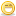# Thread: Test case for Calculator

1. ## Test case for Calculator

How many test case can you write for Calculator? Can we use BVA for this? what are the test cases?Reply With Quote

2. ## Re: Test case for Calculator

No one here is interested to write test cases for calculator because it is not one hour task, it's more than a day task.Still not sure it will complete.

So, plz try yourself friend, here members can help you if any problem is there.But no one is here to give you spoon feed.Reply With Quote

3. ##Re: Test case for Calculator

I have got all the time in the worldHere i go..

1) Test all the basic functionality +,-,*,/ . Results should be as accepted.

2) Check other complex functionality like sqrt for both +ve and -ve Numbers.

3) Divide by Zero ..Most popular test case4) Few expressions like 2+-3 .. -3+-3 .. -3-3 ..Must be tested.

5) Perform some operation (+,*..etc) number which will fill up the screen.

6) = button must be tested.

7) Screen should clear up on pressing AC.

8) Multiply by Zero.

9) Multiplication of two negative numbers must be positive. (-3*-3 = 9)

hope this will be helpful...Cheers
Vinay H MReply With Quote

4. ##Re: Test case for CalculatorOriginally Posted by vinayhmI have got all the time in the worldHere i go..

1) Test all the basic functionality +,-,*,/ . Results should be as accepted.

2) Check other complex functionality like sqrt for both +ve and -ve Numbers.

3) Divide by Zero ..Most popular test case4) Few expressions like 2+-3 .. -3+-3 .. -3-3 ..Must be tested.

5) Perform some operation (+,*..etc) number which will fill up the screen.

6) = button must be tested.

7) Screen should clear up on pressing AC.

8) Multiply by Zero.

9) Multiplication of two negative numbers must be positive. (-3*-3 = 9)

hope this will be helpful...Cheers
Vinay H M
Hi, do you think you have done all. It's not even 2% of 100 test cases. Think again before commitment. enjoy []Reply With Quote

####Posting Permissions

• You may not post new threads
• You may not post replies
• You may not post attachments
• You may not edit your posts
•